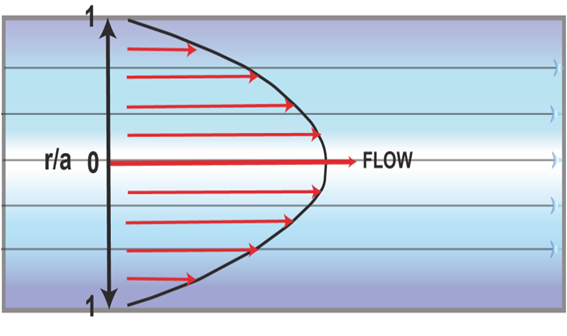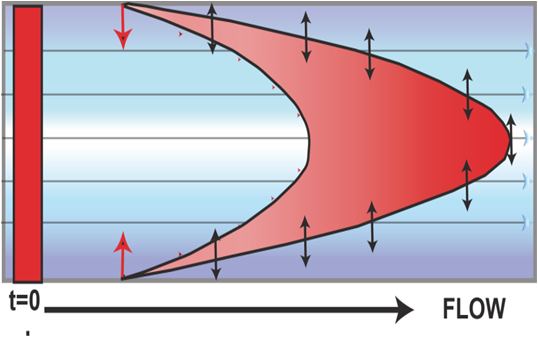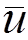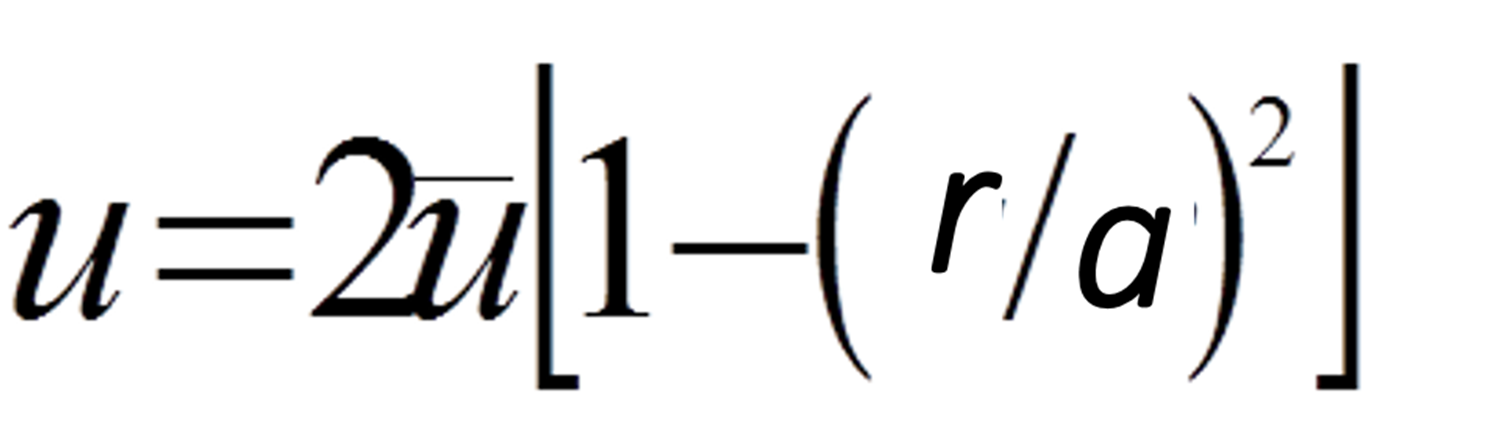0.2.2.
Straight Open Pipes
Briefly, rigorous treatment of solute dispersion in an open, straight circular tube considers the relation between axial and radial dispersion on the shape of a sample plug  as it moves downstream at a constant flow rate at a laminar flow conditions.AUnder laminar flow conditions all fluid elements follow streamlines parallel to the direction of flow and the velocity profile has a parabolic shape (A). At this, Poisseile flow, the velocity u at the tube walls is zero and it reaches is maximum value at the centre of the tube where r=0. The flow velocity u at any coordinate is:B
where r is the radial distance and a is the radius of
the channel, while     is mean flow velocity.Therefore a bolus, of initially square profile, injected at t=0, will disperse  along the velocity profile, forming a hollow, bullet shaped, three dimensional concentration gradient (B). While traveling through a detector* the gradient will yield a a sharp, asymmetric RTD curve with a long tailing section. caused by the slow movement of streamlines adjacent to the walls.

* Fast responding, with minimum volume and  monitoring perpendicularly through the passing gradient